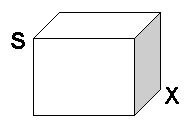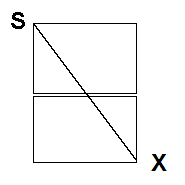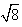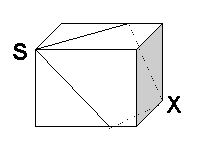The Ant on the Box Dragon

In a collection of mathematical puzzles there is this little gem of a puzzle:
A rectangular box has sides 2x1x1. A tiny ant sets out from a vertex on the surface of the box for the most distant point on the surface. Where is this most distant point? And what is its distance from the start point ?We call the start point S, and label the vertex “opposite” as X. And inspired by the puzzle, here is a neat little Dragon – a little question that needs only a Yes/No answer.
Restricting oneself to paths on the surface of the box, is X the most distant point on the surface of the box from S ?
Most people rapidly posit the truth of this conjecture. But how to really justify a gut feeling? You need to call upon those basic notions of mathematics, what cognitive scholars call mathematical heuristics, and seek to apply them.
To aid you in this task, to help you more fully grasp the conjecture, I’ve organised the following dialogue discussion of The 2x1x1 Dragon.

Mathematicus:

“In three dimensional space, the vertex X is as far away as it is possible to get from S within the solid box. And this clearly will also be the case for travel on the surface – the shortest route to the furthest point on the box will be the furthest distance one can go from the start vertex on the sides of the box.

And to work out this furthest distance, we can just flatten the sides like so:On this flattened surface the ant will travel in a straight line from S to X.
So the furthest distance is just.

Actually one can set out from S to X in two different ways - there are actually two different shortest routes – but both of length– to the most distant point on the box from S.
Like so:On this flattened surface the ant will travel in a straight line from S to X. So the furthest distance is just. Actually one can set out from from S to X in two different ways – there are actually two different shortest routes – but both of length– to the most distant point on the box from S. Like so: Here we indicate the path on a hidden face by a dashed line.
Empiricus: But you don’t even consider paths that go along that 1x1 face “opposite” S -- (shaded in the figure). This conjecture looks to me like a hard problem. The only thing I’m confident of is that the ant’s route will be made up of straight-line segments on the sides of the boxes.. I’ll just code it up in AMPLä as an optimization problem, and see just what the maximum distance is, and whether X is in fact the furthest point on the surface from S.
Mathematicus: Surely you’d be confident that for a cube, the furthest away point via the surface is the opposite vertex – opposite that is through the centre of symmetry. So by continuity, even as one stretches in one dimension, the furthest point stays where it was.
Empiricus: O.K. I’ll write a more general AMPLä program, and check out various edge ratio’s for what do you call it ? – a parallelepiped ?
Mathematicus: O ye of Little Faith. Use human reason, and not thy Computer. And a parallelepiped figure is a box each of whose faces is a parallelogram – lets stick to a box where each face is a rectangle.

Note added 2014: AMPLA now stands for any algebraic graphic program up to the task.

Those for whom this discussion is quite trivial can work out the ant's path for the case(s) where there is a hole in the box.

HAC